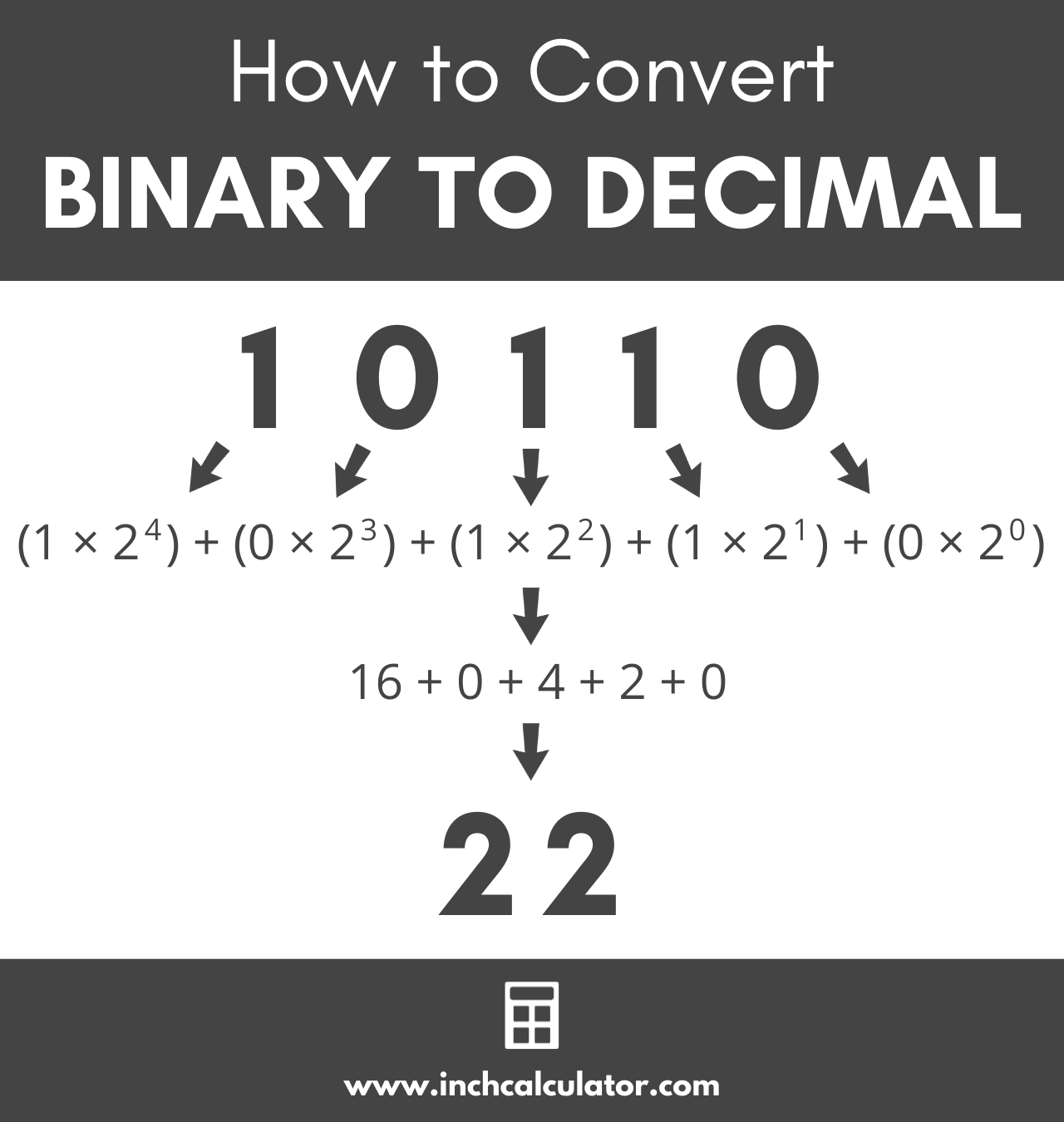# Binary to Decimal Converter

Enter a binary number below to convert it to a decimal.

## Decimal Number:

### Steps to Convert to Decimal

Learn how we calculated this below

## How to Convert Binary to Decimal

The binary number system is a base 2 number system since it only uses the digits 0 and 1. On the other hand, decimal is a base 10 number system since it uses ten digits, 0 to 9.

Often you’ll need to convert a binary number to its decimal value since most people use the decimal system. Binary numbers are often used in computing applications.

To convert a binary number to a decimal, you can use the positional notation method. To use this method, multiply each digit in the binary number from the rightmost number to the left by 2 to the power of n, where n is the distance from the right.

So, reading the binary number from right to left, the furthest digit to the right is equal to the digit times 20. The digit that is one position from the right is equal to the digit times 21.

### Binary to Decimal Formula

Thus, the binary to decimal formula is:

decimal number10 = (d0 × 20) + (d1 × 21) + … + (dn – 1 × 2n – 1)

In this formula, d0 is the binary digit furthest to the right, d1 is the digit one position from the right, and dn – 1 is the digit furthest to the left.You can also use a tool like our binary calculator to convert to decimal or hex.

For Example, let’s convert the binary number 10110 to decimal.

decimal number10 = (1 × 24) + (0 × 23) + (1 × 22) + (1 × 21) + (0 × 20)
decimal number10 = 22

## How to Convert Fractional Binary Numbers to Decimal

For real numbers that contain fractional values, you can use the positional notation system to convert as well. Start by using the method above to convert the whole portion of the number from binary to decimal.

Then, for the remaining fractional value, multiply each number to the right of the decimal point by 2 to the power of -1 times the distance from the decimal point plus 1. Ok, that sounds complicated but it’s easier than it sounds, let’s demonstrate.

decimal number10 = (d-1 × 2-1) + (d-2 × 2-2) + … + (d-n × 2-n)

For Example, let’s convert the binary number 0.101 to decimal.

decimal number10 = (1 × 2-1) + (0 × 2-2) + (1 × 2-3)
decimal number10 = (1 ÷ 21) + (0 ÷ 22) + (1 ÷ 23)
decimal number10 = 0.5 + 0 + 0.125
decimal number10 = 0.625

The calculator above can convert binary numbers to decimal, including fractional numbers like this one, along with negative numbers.

## Binary to Decimal Conversion Table

The table below shows binary numbers and the equivalent decimal number values.

Binary numbers converted to the equivalent decimal values
Binary Number Decimal Number
0 0
1 1
10 2
11 3
100 4
101 5
110 6
111 7
1000 8
1001 9
1010 10
1011 11
1100 12
1101 13
1110 14
1111 15
10000 16
10001 17
10010 18
10011 19
10100 20
10101 21
10110 22
10111 23
11000 24
11001 25
11010 26
11011 27
11100 28
11101 29
11110 30
11111 31
100000 32
1000000 64
10000000 128
100000000 256
1000000000 512
10000000000 1024
100000000000 2048